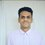# Inequality challenge proof day 2

I.. $$A,B,C$$ are angles of a triangle. Prove that

$\sum_{\mathrm{cyc}}\cos A\leq\sum_{\mathrm{cyc}}\sin\left(\frac{A}{2}\right)$

ii. Consider $a,b,c>0, abc=1$. Prove that

$\sum_{\mathrm{cyc}} \frac{a^{2}+b^{2}}{a^{8}+b^{8}}\leq a^{3}+b^{3}+c^{3}$

iii. For $a,b>0$, prove that $(1+a)^{8}+(1+b)^{8}\geq 128ab(a+b)^{2}$

iv. $p,q,r$ are distinct prime numbers such that $rp^{3}+p^{2}+p=2rq^{2}+q^{2}+q$. Find all possible values of $pqr$.

v. If $a,b$ are integers, then prove that at least one of these expressions is an integer: $\frac{a^{2}+b}{b^{2}-a},\frac{b^{2}+a}{a^{2}-b}$.

vi. $\sum_{cyclic}^{a,b,c}\frac{(b+c)(a^{4}-(bc)^{2})}{ab+2bc+ac}\geq0$.5 years, 10 months ago

This discussion board is a place to discuss our Daily Challenges and the math and science related to those challenges. Explanations are more than just a solution — they should explain the steps and thinking strategies that you used to obtain the solution. Comments should further the discussion of math and science.

When posting on Brilliant:

• Use the emojis to react to an explanation, whether you're congratulating a job well done , or just really confused .
• Ask specific questions about the challenge or the steps in somebody's explanation. Well-posed questions can add a lot to the discussion, but posting "I don't understand!" doesn't help anyone.
• Try to contribute something new to the discussion, whether it is an extension, generalization or other idea related to the challenge.

MarkdownAppears as
*italics* or _italics_ italics
**bold** or __bold__ bold
- bulleted- list
• bulleted
• list
1. numbered2. list
1. numbered
2. list
Note: you must add a full line of space before and after lists for them to show up correctly
paragraph 1paragraph 2

paragraph 1

paragraph 2

[example link](https://brilliant.org)example link
> This is a quote
This is a quote
    # I indented these lines
# 4 spaces, and now they show
# up as a code block.

print "hello world"
# I indented these lines
# 4 spaces, and now they show
# up as a code block.

print "hello world"
MathAppears as
Remember to wrap math in $$ ... $$ or $ ... $ to ensure proper formatting.
2 \times 3 $2 \times 3$
2^{34} $2^{34}$
a_{i-1} $a_{i-1}$
\frac{2}{3} $\frac{2}{3}$
\sqrt{2} $\sqrt{2}$
\sum_{i=1}^3 $\sum_{i=1}^3$
\sin \theta $\sin \theta$
\boxed{123} $\boxed{123}$

Sort by:

q3)

$(1+a)^8+(1+b)^8\ge (2\sqrt{a})^8+(2\sqrt{b})^8=256(a^4+b^4)$ It remains to prove $256(a^4+b^4)\ge 128ab(a+b)^2$ which is obvious by expansion.

- 5 years, 10 months ago

Q1) $\cos A + \cos B = 2 \cos \left(\dfrac{A+B}{2} \right) \cos \left(\dfrac{A-B}{2} \right)$

Since $A,B,C$ are angles of triangle. This gives us that $A+B+C= \pi \implies A+B = \pi - C$. We also have $\cos \left(\dfrac{A-B}{2} \right) \leq 1$

So, \begin{aligned} \cos A + \cos B &= 2 \cos \left(\dfrac{\pi-C}{2} \right) \cos \left(\dfrac{A-B}{2} \right) \\ &= 2 \sin \dfrac{C}{2} \cos \left(\dfrac{A-B}{2} \right) \\ & \leq 2\sin \dfrac{C}{2} \end{aligned}

Similarly we get $\cos B + \cos C \leq 2\sin \dfrac{A}{2}$ and $\cos C + \cos A \leq 2\sin \dfrac{B}{2}$.

$\sum_{cyc} \cos A \leq \sum_{cyc} \sin \dfrac{A}{2}$

And equality holds when $A=B=C = \dfrac{\pi}{3}$.

- 5 years, 10 months ago

What does question 4 ask for?

- 5 years, 10 months ago

Its correct now.

- 5 years, 10 months ago